# 02. Applying the Impulse-Momentum Relation

$$\newcommand{\vecs}{\overset { \rightharpoonup} {\mathbf{#1}} }$$ $$\newcommand{\vecd}{\overset{-\!-\!\rightharpoonup}{\vphantom{a}\smash {#1}}}$$$$\newcommand{\id}{\mathrm{id}}$$ $$\newcommand{\Span}{\mathrm{span}}$$ $$\newcommand{\kernel}{\mathrm{null}\,}$$ $$\newcommand{\range}{\mathrm{range}\,}$$ $$\newcommand{\RealPart}{\mathrm{Re}}$$ $$\newcommand{\ImaginaryPart}{\mathrm{Im}}$$ $$\newcommand{\Argument}{\mathrm{Arg}}$$ $$\newcommand{\norm}{\| #1 \|}$$ $$\newcommand{\inner}{\langle #1, #2 \rangle}$$ $$\newcommand{\Span}{\mathrm{span}}$$ $$\newcommand{\id}{\mathrm{id}}$$ $$\newcommand{\Span}{\mathrm{span}}$$ $$\newcommand{\kernel}{\mathrm{null}\,}$$ $$\newcommand{\range}{\mathrm{range}\,}$$ $$\newcommand{\RealPart}{\mathrm{Re}}$$ $$\newcommand{\ImaginaryPart}{\mathrm{Im}}$$ $$\newcommand{\Argument}{\mathrm{Arg}}$$ $$\newcommand{\norm}{\| #1 \|}$$ $$\newcommand{\inner}{\langle #1, #2 \rangle}$$ $$\newcommand{\Span}{\mathrm{span}}$$$$\newcommand{\AA}{\unicode[.8,0]{x212B}}$$

# Applying the Impulse-Momentum Relation

Let's re-examine the same situation we examined at the beginning of the previous chapter, a rocket launched directly upward with a time-dependent thrust.

A 2.0 kg toy rocket is fitted with an engine that provides a thrust roughly modeled by the function F(t) = (60 N/s) t - (15 N/s2) t2, for 0 < t < 4.0 s, and zero thereafter. The rocket is launched directly upward.

For analysis, we'll apply the impulse-momentum relation between:

Event 1: The instant the rocket leaves the launch-pad

Event 2: The instant the thrust drops to zero.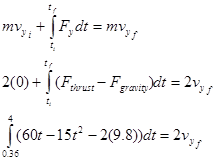Remember from last chapter that the rocket does not leave the launch pad until 0.36 s after the engine is ignited.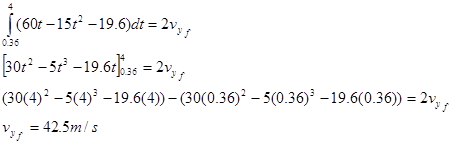When the engine shuts off, the rocket is traveling at 42.5 m/s upward.

We could also apply the impulse-momentum relation between:

Event 1: The instant the thrust drops to zero.

Event 2: The instant the rocket reaches its maximum height.

During this interval, the only force acting on the rocket is the force of gravity, and the impulse-momentum relation is:Thus, the rocket reaches its highest altitude 8.34 s after launch.

It's important to note that when a force is a function of time, it's relatively easy to integrate the function and determine the impulse. However, it should be clear that it would not be easy to determine the work done by a force of this type. Since work is expressed as an integral of a force with respect to a displacement (dr), the force function has to be expressed in terms of position, r. In general, it's not an easy (or sometimes possible) task to "convert" a function of time into a function of position, so work-energy is not a particularly useful way to analyze systems when the forces acting are time dependent. However, if the forces depend upon the position of the object, work-energy is a powerful analysis tool.

# Applying the Work-Energy Relation

A 0.15 kg ball is launched vertically upward by means of a spring-loaded plunger, pulled back 8.0 cm and released. It requires a force of about 10 N to push the plunger back 8.0 cm.

## The Force Exerted by a SpringThe force that the spring exerts on the ball depends on the amount by which the spring is compressed. The more the spring is compressed, the larger the force it exerts on the ball. A common model is that the force exerted by a spring is directly proportional to the amount of deformation of the spring, in this case compression. Deformation (s) is defined to be the difference between the current length of the spring (L) and the equilibrium length (L0):If we define the positive coordinate direction to point in the same direction as positive deformation (stretch), then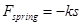with the proportionality constant, k, referred to as the spring constant.

For the plunger, since it takes 10 N to compress the spring by 8.0 cm,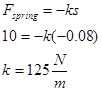For analysis, we'll apply the work-energy relation between:

Event 1: The instant the plunger is released.

Event 2: The instant the ball reaches its maximum height.

For these two events, work-energy looks like this: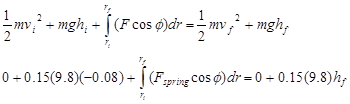To do the integral, we must express Fspring in terms of the variable of integration, r. For the coordinate system chosen, s and r are identical. Also note that the force of the spring and the direction of motion of the ball point in the same direction. Thus, f = 0.The ball reaches a maximum height of 19 cm above the top of the plunger.

This page titled 02. Applying the Impulse-Momentum Relation is shared under a CC BY-NC-SA license and was authored, remixed, and/or curated by Paul D'Alessandris.